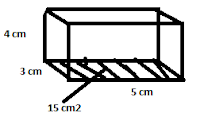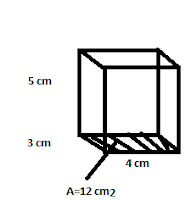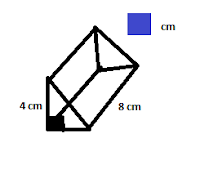## Tuesday, February 15, 2011

### Nikki's Volume Scribe Post2.

5. a)
Determine the volume of each right rectangular prism.

LxWxH LxWxH
3x4=12cm3 3x4=12 cm3
12x5=60 cm3 12x5=60cm3

8. Nina uses 15 centimeter cubes to make the base of the rectangular prism. Determine the volume if the prism has a total of 5 layers of cubes. Show your thinking.

If there are 5 layers of 15 cm cubes you times 15x5 which equals 75 cm3
5x15=75 cm3

10. A water through is in a shape of a right triangular prism with base area of 1250 cm2 and a height of 100 cm. How much water can be put in there before it overflows?

1250x100=125 000 cm3

Here is 1 question from 7.3 on page 265-267

4. Determine the volume of each cylinder.
a)h=23 cm
r=5 cm
d=2.r
d=5.2
d=10 cm
C=Pi.d
C=3.14x10
C=31.4
v=1805.5 cm3

b)r=14 cm
h=12 cm
d=r.2
d=14.2
d=28 cm
C=pi.d
C=3.14.28
C=87.92
V=7385.28 cm3

c)r=0.5 m
h=1.5
d=r.2
d=0.5.2
d=1 m
C=pi.d
C=3.14.1
C=3.14
V=1.177

9. The Canadarm has a cylinder called a capture envelope that is used to catch objects floating in space. It is 20.3 cm in diameter and 10 cm deep. What is the maximum volume it can hold?

7.4 Page 275
18. The volume of the triangular prism shown is 48 cm3. What is the volume of the missing measurement.5 cm3